# independent set

## The Double Cap Conjecture ★★

Author(s): Kalai

Conjecture   The largest measure of a Lebesgue measurable subset of the unit sphere ofcontaining no pair of orthogonal vectors is attained by two open caps of geodesic radius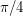around the north and south poles.

## Hitting every large maximal clique with a stable set ★★

Author(s): King; Rabern

Conjecture   There is a universal constantsuch that every graph contains a stable set which intersects every maximal clique of size.

Conjecture   Every graph contains a stable set which intersects every maximal clique of size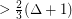.

Keywords: independent set; maximal clique

## Aharoni-Berger conjecture ★★★

Author(s): Aharoni; Berger

Conjecture   If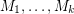are matroids on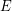andfor every partition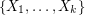of, then there existswithwhich is independent in every.

Keywords: independent set; matroid; partition